This is the content of a presentation of F# that I will have at the office in a couple of weeks. I wrote this presentation in HTML using the S5 framework by Eric Meyer.

F# is a programming language that allows you to write simple code to solve complex problems.

Don Syme

The quote comes from Don Syme.

With programming language, Don means that F# is not a new platform, but just another language on the CLR. This means that the libraries that already works for C# and VB.NET are likely to also work well with F#.

One strength with F# is to express as much intent as possible with little code. The theory is that quantity of code cause bugs and if we could limit the amount code we would also limit the amount of bugs. Reports from users has also told stories about exceptionally small amount of bugs in code produced in F#. This might be a hard statement to prove since F# draws the attention of developers of a different kind.

Don Syme says that F# is not a language meant to solve all problems, but specific problems of a complex nature. F# has never meant to replace C# or VB and is not suitable for tasks that depends on changing a mutable state. The first thing that comes to mind is Workflows and state machines.

## Functional .NET

### Two paradigms that rule the F# language

1. Everything is a function
2. Everything is immutable

Since every good thing comes in trees we should specify the big threes for functional programming.

We will look at what a function is in F# and how treating everything as a function changes the way you code.

If you define a variable in F# it is immutable by default which means that its value will never change. This changes the way you loop and aggregate things in F# compared to an imperative language like C#.

## Getting Started

### F# Interactive is found in View/Other Windows/F# Interactive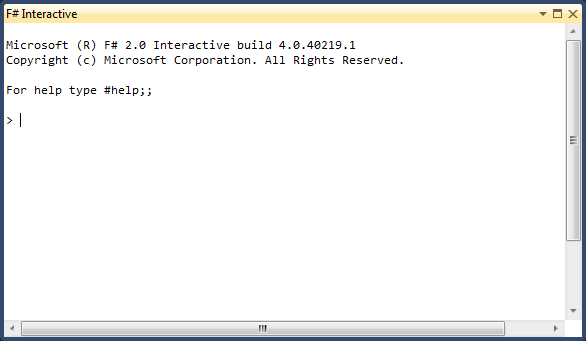You will find the F# interactive window in the View/Other Window menu option. This is where you can evaluate your expressions as you code. Simply copy the code to the interactive and add double semicolon ';;' to evaluate, or use one of the shortcut commands in Visual Studio. Mine is, mark the code to evaluate and press Alt + '

## Everything is a function

``````let area w h = w * h
val area : int -> int -> int
``````
``````let half x = x / 2
val half : int -> int
``````
``````let triangleArea w h = half (area w h)
val triangleArea : int -> int -> int
``````
``````let myTriangle = triangleArea 2 4
val myTriangle : int = 4
``````

A function is defined with the keyword let. First argument is the name of the function and the rest are arguments to that function. After the "=" (equals sign) comes the function body. In F# we don't make a distinction between variables and functions with no arguments. These are the same. If the expression can be evaluated it will.

Argument types are inferred at compile time. Sometimes the compiler can't inferr the types and we'll have to specify them explicity.

## Mutability

This imperative language uses the side effect of the for loop to change the mutable state of the result variable.

```namespace LiteMedia.CSharpLecture
{
public class Example1
{
const int Max = 100000;

public void Aggregate()
{
var result = 0;
for (int i = 1; i < Max; i++)
result += i;

System.Console.WriteLine(result);
}
}
}```

If the world can't change it won't have side effects. Since the world can't change we continue to create new and better versions of the world.

Imperative programming languages depends on changing states of the program. This is why you aggregate by adding numbers to a result variable.

## Immutability → Purity

In a functional programming language where variables are immutable, state won't change.

```let sum max =
let result = 0
for i = 0 to max do
result <- result + i
result

sum 100000```

``````Result: error FS0027: This value is not mutable
``````

Clearly, this program does not work as intended.

You can't change the state of an immutable variable. This means that

• Traditional looping makes little sense in F# - recursion
• Output of a function depends only on the input arguments - purity
• Side effects are eliminated
• Calling function f(x) twice will yield the same result both times

## Immutability → recursion

```let rec sum max =
if max = 0 then
0
else
max + sum (max - 1)```

### Function calls

``````sum 3 = 3 + (sum 2)
sum 2 = 2 + (sum 1)
sum 1 = 1 + (sum 0)
sum 0 = 0
3 + 2 + 1 + 0 = 6
``````

Since we can't change the value we will have to create a new value, and easiest way of doing that is calling the method again with different arguments. This is called recursion.

## Recursion in F

Doing it for real does not involve if statements

```let rec sum max =
match max with
| 0 -> 0
| _ -> max + sum (max - 1)```

match..with is such common operation it has an alias: `function`

```let rec sum = function
| 0 -> 0
| n -> n + sum (n - 1)```

Recursion is not done in F# with if statements, but with matching patterns. This works pretty much like a switch statement on steroids.

## Aggregation

``````let sum max = [1..max] |> List.fold (+) 0
``````

could be written in C#

``````var result = Enumerable.Range(1, Max).Aggregate((acc, x) => acc + x);
``````

yielding numbers in F#

``````let sum max = seq { for i in 1..max do yield i } |> Seq.fold (+) 0
``````

Recursing to sum up all the digits from 1-100000 is quite unnessesary. This is how you would do it by using a list, and F# built in Fold.

You can accomplish the same thing with Linq.Aggregate.

Since Linq.Aggregate yields numbers as we request them, this is a more effective solution. The F# code has to first create the list and then sum it up. We can mend this by also yielding numbers.

Even though, the F# solution is 66 characters and the C# solution is 72.

## Operators are functions

``````(+)
val it : (int -> int -> int)
``````
``````(*) 6 7
val it : int = 42
``````
``````let (++) a b = (a + b) * 2
5 ++ 7
val ( ++ ) : int -> int -> int
val it : int = 24
``````

Operators are functions too. Just evaluating the + operator will tell us that it is a function that takes two integers and returns an integer. We can use it as a function with prefix notation as well as the more ordinary infix notation.

Creating our own custom operators is trivial, just like defining any function and can be used with both prefix and infix notation.

## Partial function calls

``````let addFive = (+) 5
val addFive : (int -> int)
``````
``````[1; 2; 4] |> List.map addFive
val it : int list = [6; 7; 9]
``````

When we call a function with less arguments we create a new function with the missing parameters as arguments.

Once we have the correct function definition we can use it anywhere. For example mapping the function onto values in a list.

## Anonymous functions

Just like lambdas in C# we have anonymous functions in F#.

``````(fun x -> x * x) 7
val it : int = 49
``````

## Functions as arguments

``````[1..10] |> List.filter (fun x -> x % 2 = 0)
val it : int list = [2; 4; 6; 8; 10]
``````

Just like in C# we have anonymous functions in F#. We use these as arguments to other functions.

For every number from 1 to 10, filter out those that are x % 2 = 0, even.

## Composite functions

### When one function is not enough..

``````type Color = | Red | Green | Blue
let colors = [Red; Red; Red]
``````

### Is there any color that is not red?

``````colors |> List.exists (fun c -> c <> Red)
``````

### Without lambda expressions

``````colors |> List.exists (not << (=) Red)
``````

### Same thing as

``````(fun c -> not ((=) Red c))
``````

We can add functions together in F#, very much like calling a function with the result from another function. We do this with the operator << or >>. That means, take the result of this function and feed it to the next function. This can be very useful for simplify things.

## NULL does not exist

### Have you ever seen this before?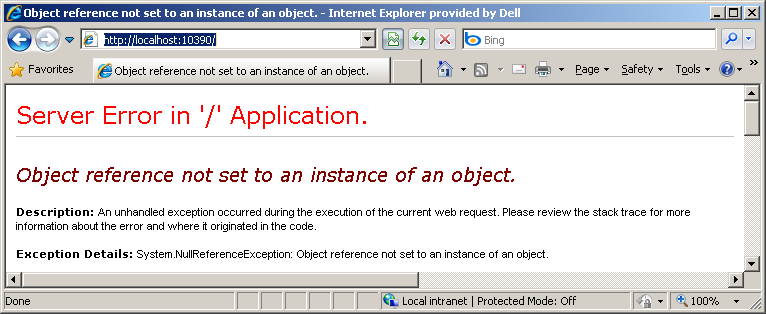Have you ever seen the null reference exception YSOD? Then you will be glad to know that no function in F# may return null.

## Option<'a>

```let rec findPrime l =
let isPrime n = [2..(n/2)]
|> List.exists (fun x -> n % x = 0) |> not

match l with
| [] -> None
| head :: tail -> findPrime tail```

### We can match on the option<int>

```let hasPrime l =
match findPrime l with
| None -> false
| Some(x) -> true

[4; 6; 8; 9; 11] |> hasPrime```

``````val it : bool = true
``````

Instead of returning null we use the new Some(x)/None functionality. This lets us match on the return value. In this example we have a function that will return first prime number in the list, or None.

We can create a hasPrime function that will check if we get Some(x) that is prime or if we get None.

### Why is Option<'a> better than NULL?

• The Option exists in the function definition. `val findPrime : int list -> int option`

• The NULL value is an indirect side effect of references. The Option is explicit. When you call the findPrime function, you have to handle the Option result.

• The match..with pattern matching is designed to handle Some/None values. ```fsharp match findPrime l with | None -> false | Some(x) -> true ```

## Records for data structures

You can define complex data structures as records

``````type Book = { Title : string; Author : string }
let book = { Title = "The Treasure Island";  Author = "Robert Lewis Stevenson" }
``````

but remember that everything is immutable

``````book.Title <- "Treasure Island"
error FS0005: This field is not mutable
``````

If you need to define more complex data structures you can define a record type. But you'll have to remember that this type is immutable as everything else. You can't change its values once it has been set.

## Records as values

You use records in functions as any other value

```type Point = { x : int; y : int }

let graph fn width height =
// Is point y between y1 and y2
// int -> int -> Point -> bool
let yBetween y1 y2 point = point.y > y1 && point.y < y2

// For all x, -100 to 100
[-(width / 2)..(width / 2)]
|> List.map (fun x -> { x = x; y = fn x})
|> List.filter (yBetween -(height / 2) (height / 2))```

In this example we would like to spot a graph on a panel.

It's nice to notice that the compiler will asume that we create a Point type at line 10, and we use the partial method yBetween to filter out points at line 11.

When I see such code, I find it amusing to think that F# is a statically typed language and yet, we don't specify types anywhere but in the type definition. The compiler will try to find out the types as we go and will tell us where it fails.

``````graph (fun x -> 2 * x + pown x 3) 200 200
val it : Point list = [{x = -4; y = -72;}; {x = -3; y = -33;}; {x = -2; y = -12;}; {x = -1; y = -3;}; {x = 0; y = 0;}; {x = 1; y = 3;}; {x = 2; y = 12;}; {x = 3; y = 33;}; {x = 4; y = 72;}]
``````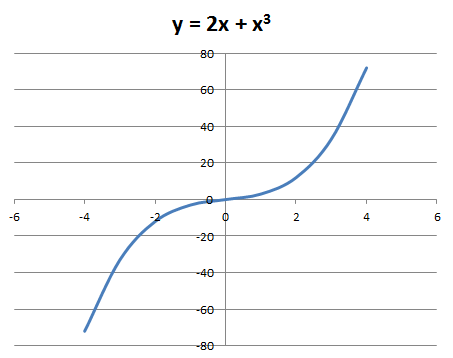The panel is 200x200 and the graph we would like to draw is y = 2x + x^3. For this purpose we use create a series of point types from x = -100 to x = 100 with the distinction that y also has to be within -100 < y < 100.

## Object orientation

### A new class called Queue

```type Queue() =
let mutable queue = []

member this.Empty = queue = []

member this.Push x = queue <- queue @ [x]

member this.Pop =
match queue with
| [] -> None
queue <- tail

You create a class very much like a record. When you want to specify member methods you use the keyword member instead of let. I use this to identify the current instance of the class.

Since object oriented programming is very much about changing states of objects, you can create mutable fields within the class. You specify the mutable keyword after let to tell F# that the field is mutable.

``````type Queue =
class
new : unit -> Queue
member Push : x:obj -> unit
member Empty : bool
member Pop : obj option
end
``````

## Using our queue object

``````let queue = new Queue()
val queue : Queue
``````
``````[1; 2; 3] |> List.iter queue.Push
val it : unit = ()
``````

```let rec dequeue (q : Queue) =
match q.Empty with
| true -> []
| false -> q.Pop.Value :: dequeue q
dequeue queue```

You create a new instance the same way you do in C# with the new keyword. We can write a function that will dequeue the whole queue into a list.

## Unit of measure

### An int is not just an int

``````[<Measure>] type m
[<Measure>] type s

let distance = 100.0<m>
let worldRecord = 9.58<s>
let speed = distance / worldRecord

val speed : float<m/s> = 10.43841336
``````
``````let km = 1000.0<m>
let h = 3600.0<s>

let speedInKmPerHour = speed / (km/h)
val it : float = 37.5782881
``````

What is an int? When I went to school we were forced to answer every math question with the unit of the answer.

-If you take two apples and add three apples, how many apples have you got?
-Five!
-Five, what?
-Five apples.

With this in mind, an int is not just an int. We usually try to tell in the variable name, what the int symbolizes but that is not very type safe. Welcome to a world of units of measure.

```// Identity monad
type Identity<'a> =
| Identity of 'a

type IdentityBuilder<'a>(v : 'a) =
let value = v
member self.Bind ((Identity v), f) = f(v)
member self.Return v = Identity v

let identity = new IdentityBuilder<int>(0)

identity { return System.Int32.Parse("42")  }```

This is an Identity Monad defined in F#. If you don't know what a monad is, you don't have to, because in F# this is abstracted into computational expressions. When a monad is defined it can be used as a computational expression as you see on line 13.

## Asyncronous F

```open System.Net
open Microsoft.FSharp.Control.WebExtensions

let webGet (name, url) =
async {
let uri = new System.Uri(url)
let webClient = new WebClient()
return name, html.Length
}

let addresses = [ "Valtech", "http://www.valtech.se";
"LiteMedia", "http://litemedia.se" ]

let contentLengths =
|> Seq.map webGet
|> Async.Parallel
|> Async.RunSynchronously```

Async in F# is a monad. That means that you write asynchronous tasks within a computational expression and bind the async monad to a async task.

webGet is a function with the signature `'a * string -> Async<'a * int>` and this enable us to run several instances of this function in parallel. There are several helper functions in F# like AsyncDownloadString that will make it easier for us to write these async tasks.

The example will get content lengths of addresses of a list, in parallel.

## Unit Testing F

### No ceremony unit testing

```open Xunit

// SUT
let add a1 a2 = a1 + a2

[<Fact>]
let result = add 8 4
Assert.Equal(12, result)```

Most exciting part of unit testing with F# is the complete lack of ceremony. All you need is an `open Xunit` and you're all set writing tests.

Notice the end parathesis of the let function. This is needed because without it F# will give a function with the definition `unit` where as Xunit will look for tests with the definition `unit -> unit`. The paranthesis forces this function signature.

## Test doubles

### Imagine the following LoginController using a repository

`404: Not Found`

Following is the system we would like to test. The problem is that we have to stub the userRepository to be able to create an instance of LoginController and test the login method. In C# I would use a framework like Rhino Mocks or Moq, but how do we tackle this problem in F#?

### Please to meet object expressions

```[<Fact>]
// Setup
let user = new User("Mikael", "Hello")

let repository = {
new IRepository<User> with
member this.GetAll() = [|user|] :> seq<User> }

// Test

// Assert
Assert.True(result)```

In F# we can generate concerete instances of any abstract class or interface with object expressions. This is very useful when creating test doubles in testdriven development, since we no longer have any use for stubbing frameworks - only mocking.

## Web development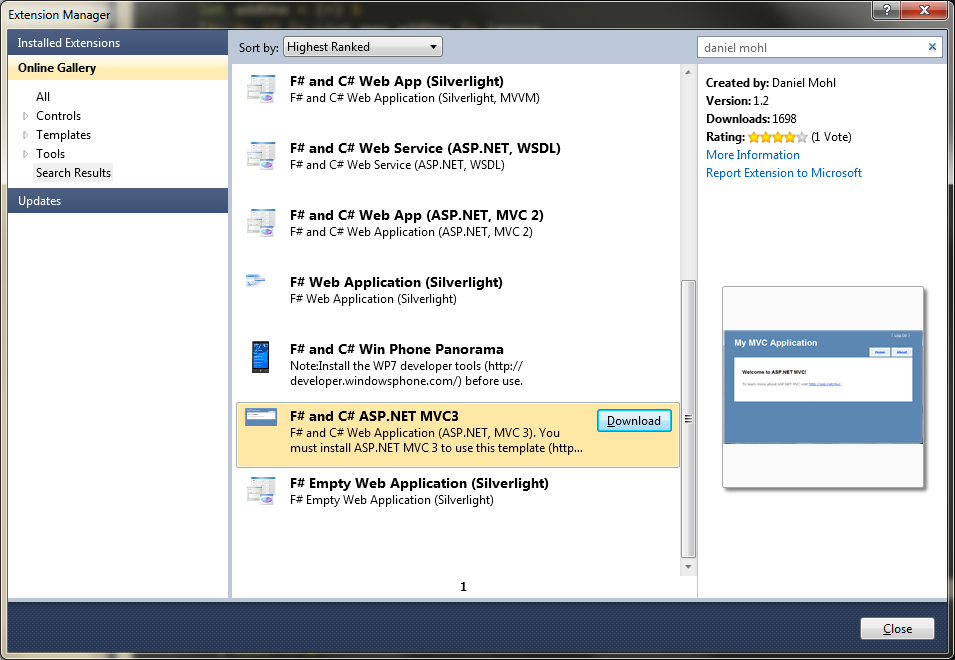Daniel Mohl has written a couple of extensions that will help you getting started with F# web development. You'll find them in the Tools/Extension Manager within Visual Studio. Select the Online tab and search for Daniel Mohl to find all of his extensions available.

### Create a new project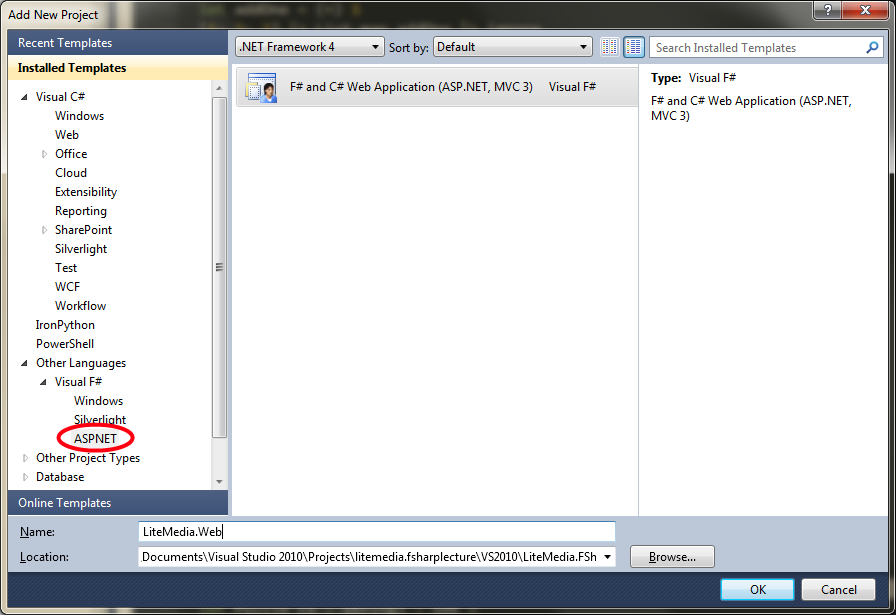With Daniel Mohl's extension installed you should be able to create a new F# ASPNET project from the Add New Project menu.

### The project stub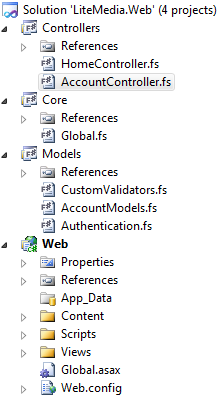The project created is the same project that you would create for a C# MVC site, but with F# libraries instead. The route setup is translated into F# as with the model binders.

### Writing our first home controller

```namespace LiteMedia.Web

open System.Web
open System.Web.Mvc

[<HandleError>]
type HomeController() =
inherit Controller()

member x.Index () : ActionResult =
x.ViewData.["Message"] <- "Welcome to ASP.NET MVC!"
x.View() :> ActionResult

x.View() :> ActionResult```

Our HomeController is very simple and it displays how to write the index and about methods. Nothing here that is suprising. Very simplistic code and yet everything that comes with the original C# version of this example site.

## WCF in F

### It's easy to define a web service in F

```[<ServiceContract(ConfigurationName = "IsItFriday",
Namespace = "http://litemedia.se/IsItFriday")>]
[<ServiceBehavior(InstanceContextMode=InstanceContextMode.PerCall)>]
type public IsItFriday() =
class
[<OperationContract>]
member public x.Query() =
DateTime.Today.DayOfWeek = DayOfWeek.Friday
end```

### Kickstart the service

``````let host = new ServiceHost( typeof<IsItFriday>, [|new Uri("http://localhost:18889/")|]);
host.Open();
``````

Writing a WCF web service in F# is pretty straight forward since web services is all about functions and not preserving state.

### The web service up an running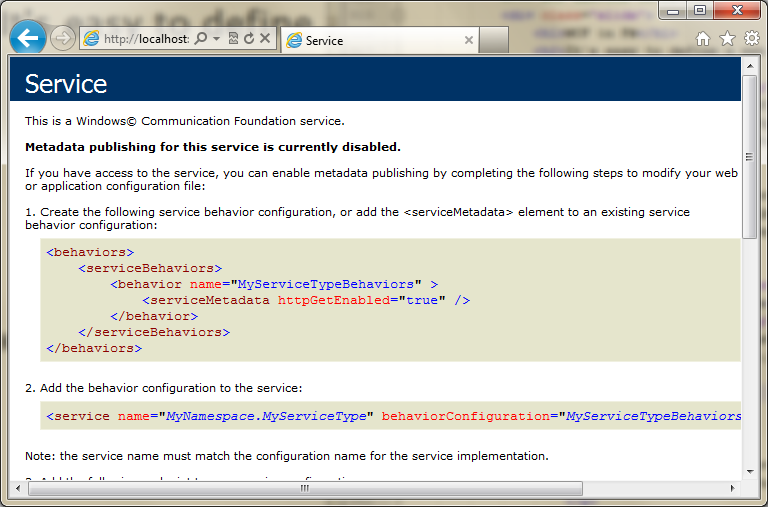## Fibonacci

```let fibonacci = Seq.initInfinite (fun i ->
let rec fibonacciinner = function
| 0 -> 0
| 1 -> 1
| n -> fibonacciinner (n - 1) + fibonacciinner (n - 2)
fibonacciinner i)```

### Result

``````val fibonacci : seq<int><br /> val it : seq<int> = seq [0; 1; 1; 2; ...]
``````

## Sieve of Eratosthenes

```let primes n =
let rec sieve = function
| [] -> []
``````primes 100